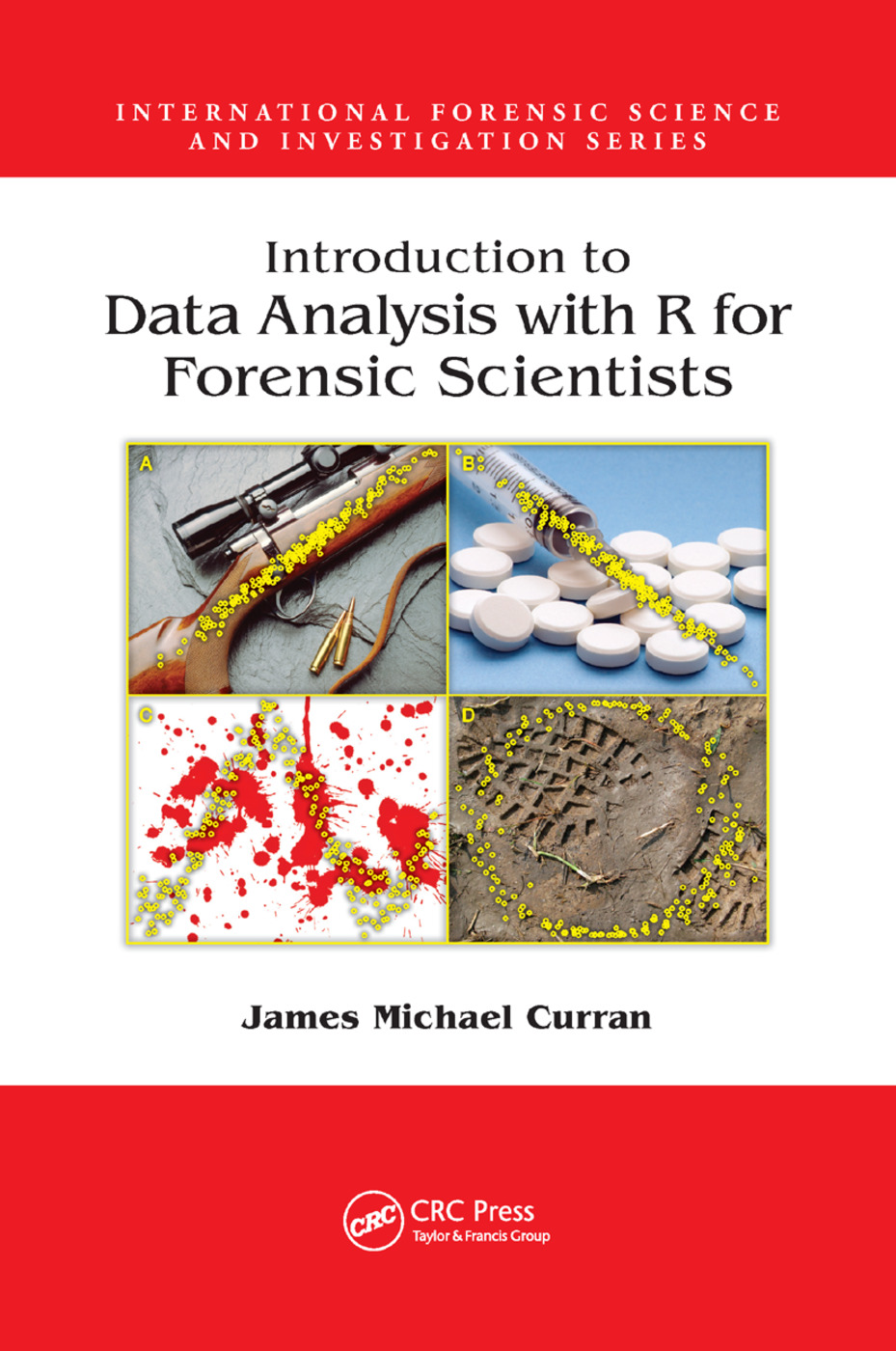Introduction to Data Analysis with R for Forensic Scientists

1st Edition

CRC Press

331 pages | 95 B/W Illus.

New in Paperback: 9781138381445
pub: 2019-06-07
\$71.00
x
Hardback: 9781420088267
pub: 2010-07-30
Currently out of stock
\$135.00
x
eBook (VitalSource) : 9780429250217
pub: 2010-07-30
from \$65.00

FREE Standard Shipping!

Description

Statistical methods provide a logical, coherent framework in which data from experimental science can be analyzed. However, many researchers lack the statistical skills or resources that would allow them to explore their data to its full potential. Introduction to Data Analysis with R for Forensic Sciences minimizes theory and mathematics and focuses on the application and practice of statistics to provide researchers with the dexterity necessary to systematically analyze data discovered from the fruits of their research.

Using traditional techniques and employing examples and tutorials with real data collected from experiments, this book presents the following critical information necessary for researchers:

• A refresher on basic statistics and an introduction to R
• Considerations and techniques for the visual display of data through graphics
• An overview of statistical hypothesis tests and the reasoning behind them
• A comprehensive guide to the use of the linear model, the foundation of most statistics encountered
• An introduction to extensions to the linear model for commonly encountered scenarios, including logistic and Poisson regression
• Instruction on how to plan and design experiments in a way that minimizes cost and maximizes the chances of finding differences that may exist

Focusing on forensic examples but useful for anyone working in a laboratory, this volume enables researchers to get the most out of their experiments by allowing them to cogently analyze the data they have collected, saving valuable time and effort.

Introduction

Who is this book for?

What this book is not about

How this book was written

Why R?

Basic statistics

Introduction

Definitions

Simple descriptive statistics

Summarizing data

The dafs package

R tutorial

Graphics

Introduction

Why are we doing this?

Flexible versus \canned"

Drawing simple graphs

Annotating and embellishing plots

R graphics tutorial

Hypothesis tests and sampling theory

Topics covered in this chapter

Statistical distributions

Introduction to statistical hypothesis testing

Tutorial

The linear model

Simple linear regression

Multiple linear regression

Calibration in the simple linear regression case

Regression with factors

Linear models for grouped data - One way ANOVA

Two way ANOVA

Unifying the linear model

Modeling count and proportion data

Introduction to GLMs

Poisson regression or Poisson GLMs

The negative binomial GLM

Logistic regression or the binomial GLM

Deviance

The design of experiments

Introduction

What is an experiment?

The components of an experiment

The principles of experimental design

The description and analysis of experiments

Fixed and random effects

Completely randomized designs

Randomized complete block designs

Designs with fewer experimental units

James M. Curran is currently an Associate Professor of Statistics in the Department of Statistics at the University of Auckland (Auckland, New Zealand). Dr. Curran is also the co-director of the New Zealand Bioinformatics Institute at the University of Auckland (www.bioinformatics.org.nz).